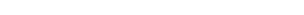xSimple shearEncyclopedia
In fluid mechanics
Fluid mechanics
Fluid mechanics is the study of fluids and the forces on them. Fluid mechanics can be divided into fluid statics, the study of fluids at rest; fluid kinematics, the study of fluids in motion; and fluid dynamics, the study of the effect of forces on fluid motion...

, simple shear is a special case of deformation
Deformation (mechanics)
Deformation in continuum mechanics is the transformation of a body from a reference configuration to a current configuration. A configuration is a set containing the positions of all particles of the body...

where only one component of velocity
Velocity
In physics, velocity is speed in a given direction. Speed describes only how fast an object is moving, whereas velocity gives both the speed and direction of the object's motion. To have a constant velocity, an object must have a constant speed and motion in a constant direction. Constant ...

vectors has a non-zero value: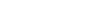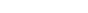In vector calculus, the gradient of a scalar field is a vector field that points in the direction of the greatest rate of increase of the scalar field, and whose magnitude is the greatest rate of change....

of velocity is constant and perpendicular to the velocity itself: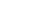,

where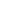is the shear rate and: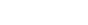The deformation gradient tensor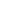for this deformation has only one non-zero term: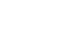Simple shear with the rateis the combination of pure shear strain with the rate of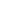and rotation
Rotation
A rotation is a circular movement of an object around a center of rotation. A three-dimensional object rotates always around an imaginary line called a rotation axis. If the axis is within the body, and passes through its center of mass the body is said to rotate upon itself, or spin. A rotation...

with the rate of:Important examples of simple shear include laminar flow through long channels of constant cross-section (Poiseuille flow), and elastomeric bearing pads in base isolation
Base isolation
Base isolation, also known as seismic base isolation or base isolation system, is one of the most popular means of protecting a structure against earthquake forces...

systems to allow critical buildings to survive earthquakes undamaged.

## Simple shear in solid mechanics

In solid mechanics, a simple shear deformation is defined as an isochoric plane deformation in which there are a set of line elements with a given reference orientation that do not change length and orientation during the deformation.

If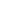is the fixed reference orientation in which line elements do not deform during the deformation and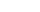is the plane of deformation, then the deformation gradient in simple shear can be expressed as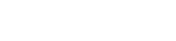We can also write the deformation gradient as